Kenneth See, ACCA

Master in Computer and Information Technology at UPenn || Research Focus in FinTech & RegTechKenneth See, ACCA

Master in Computer and Information Technology at UPenn || Research Focus in FinTech & RegTech

Published  Mar 27, 2021
Principal Components Analysis is an interesting tool that can be used for stock market analysis. Using Python, it is even possible to use it without diving too deep into the mathematics.Principal Components Analysis (PCA) is a statistical process that helps summarize data sets with a large number of variables into smaller sets of principal components. This is a powerful method for visualizing clusters and performing dimension reduction.

In this article, I will be demonstrating how PCA can be used in analyzing the stock market.

How PCA works

The PCA process can be summarized into the following 4 steps:

1. Standardize the data
2. Compute the covariance matrix
3. Find the eigenvalues and eigenvectors for the covariance matrix
4. Compute the orthogonal projection matrix and project the data onto the subspace spanned by the eigenvectors

Understandably, this may sound alien to anybody who has not taken a linear algebra class. Thankfully, the scikit-learn Python package abstracts all these away and gives us a way to perform PCA without diving into the nitty-gritty matrix computations.

Preparing the data

I used the daily returns of composite stocks of the Dow Jones Industrial Average (DJIA) for my analysis. This index was selected because there are only 30 stocks to work with, making it easier for demonstration, and still rather diverse in terms of sector representation.

As always, I imported the necessary libraries.

``````import numpy as np
import pandas as pd
import requests
import json
import time
import matplotlib.pyplot as plt
from datetime import datetime
from sklearn.preprocessing import StandardScaler
from sklearn.decomposition import PCA``````

I also created the function to retrieve financial data from the AlphaVantage API as shown in my previous article.

``````def retrieve_data(function: str, symbol: str, api_key: str) -> dict:
"""
Retrieves data from AlphaVantage's open API.
Documentation located at: https://www.alphavantage.co/documentation
"""
# query from API
url = f'https://www.alphavantage.co/query?function={function}&symbol={symbol}&apikey={api_key}'
response = requests.get(url)
data = response.text
# parse output

return parsed``````

Using the function, I queried for the SPDR Dow Jones Industrial Average ETF Trust (ticker symbol: DIA), which is an ETF that tracks the DJIA. I would need the daily close prices to compute the daily returns, so upon inspecting the data returned from the API call, I identified that the information I needed was in the "Time Series (Daily)" key.

``````index = 'DIA'
price_json = retrieve_data('TIME_SERIES_DAILY', index, api_key)``````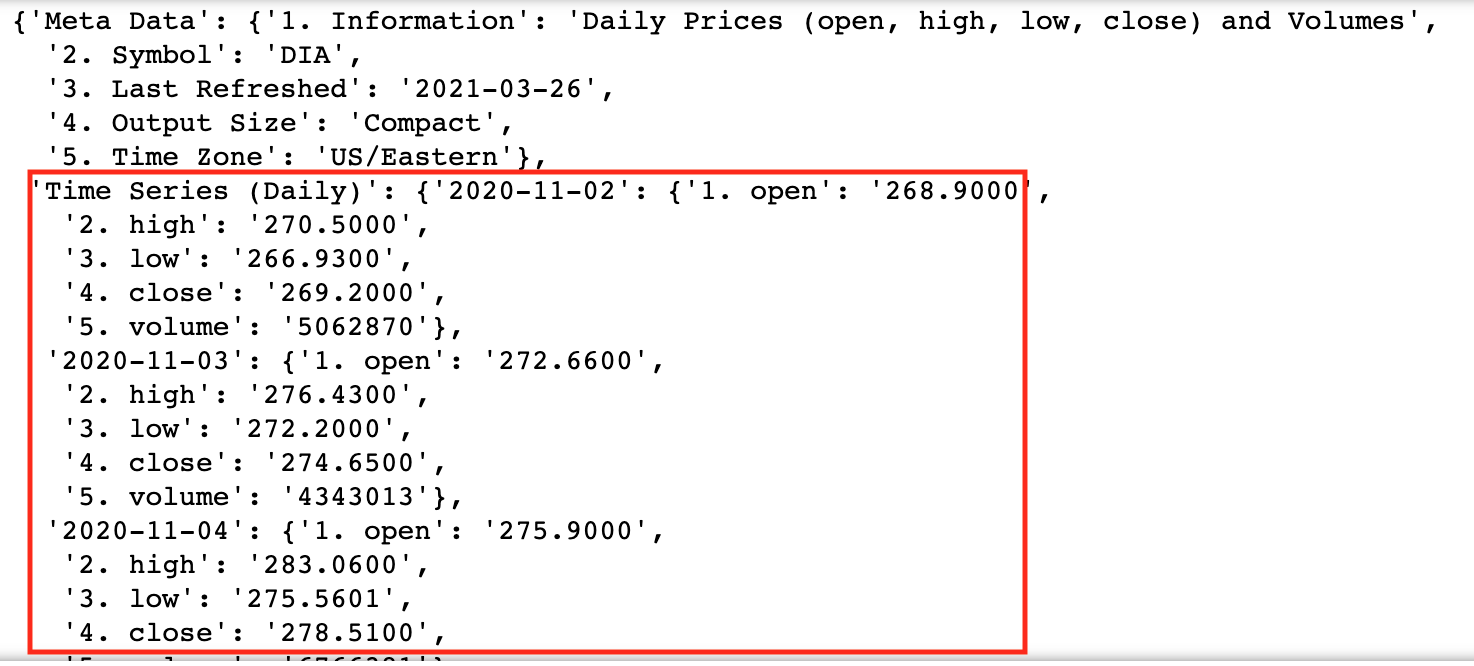I could then extract the data I needed by iterating through the list of "Time Series (Daily)" and then loading the extracted data into a Pandas data frame.

``````# extract relevant data
dates = []
close_price = []
for date, data in price_json['Time Series (Daily)'].items():
dates.append(datetime.strptime(date, '%Y-%m-%d'))
close_price.append(float(data['4. close']))

# load relevant data into data frame
df_ts = pd.DataFrame({'Date': dates, 'Close_Price': close_price})``````

Similar to my previous article on computing the Relative Strength Index, I could get the previous close price for each day by copying the close price column and shifting them up by a row. Using the day's close price and previous close price, I could then compute the daily return as (close price / previous close price) - 1 and load it into a new data frame.

``````# calculate daily return
df_ts['Previous_Close_Price'] = df_ts['Close_Price'].shift(periods=-1)
df_ts['Daily_Return'] = df_ts['Close_Price']/df_ts['Previous_Close_Price'] - 1

# load daily return into new data frame
df_daily_returns = pd.DataFrame(data=df_ts['Daily_Return'].tolist(), columns=[index])``````

Next, I had to obtain the daily returns for the DJIA composite stocks. I had created a CSV file that contained a list of the composite stocks, as well as their ticker and sector information, so I read the file into a data frame.

``DJI = pd.read_csv('DJI_composition.csv')``

I could just iterate through each stock symbol and follow similar steps as the DIA fund to obtain and prepare the daily return data for each stock.

``````DJI_symbols = DJI['Symbol'].tolist()

# iterate through each symbol
for symbol in DJI_symbols:
try:
price_json = retrieve_data('TIME_SERIES_DAILY', symbol, api_key)

# extract relevant data
dates = []
close_price = []
for date, data in price_json['Time Series (Daily)'].items():
dates.append(datetime.strptime(date, '%Y-%m-%d'))
close_price.append(float(data['4. close']))

# load relevant data into data frame
df_ts = pd.DataFrame({'Date': dates, 'Close_Price': close_price})

# calculate daily return
df_ts['Previous_Close_Price'] = df_ts['Close_Price'].shift(periods=-1)
df_ts['Daily_Return'] = df_ts['Close_Price']/df_ts['Previous_Close_Price'] - 1

df_daily_returns[symbol] = df_ts['Daily_Return'].tolist()

time.sleep(15) # to handle limited number of calls permitted in AlphaVantage's free tier
except:

Lastly, I dropped the last row in the data frame which contained NA values, and transposed the data frame. The transposing is to ensure that each row represented the returns for a stock.

``````df_daily_returns.dropna(inplace=True)
df_daily_returns_trans = df_daily_returns.transpose()``````

Finding the principal components

From the transposed data frame, I extracted only the returns of the composite stocks and standardized them using the StandardScaler function from the preprocessing package in scikit-learn.

``````x = df_daily_returns_trans.loc[DJI_symbols, :].values
x = StandardScaler().fit_transform(x)``````

With the help of the decomposition package in scikit-learn, I could simply fit the standardized data into the PCA class to obtain the principal components. For this case, I would only need to get 2 principal components but it is possible to specify more or less.

``````pca = PCA(n_components=2)
components = pca.fit_transform(x)``````

The principal components were then loaded into a data frame and the output looks something like this:

``````df_components = pd.DataFrame(
data=components,
columns=['pc1', 'pc2']
)``````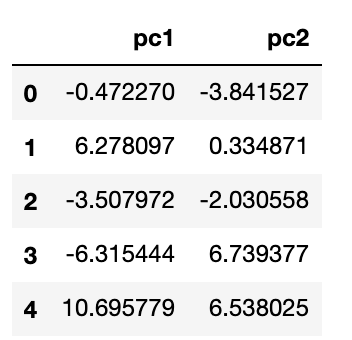Visualizing clustering

We could do a quick visualization by plotting the principal components against each other in a scatter plot.

``df_components.plot.scatter(x='pc1', y='pc2')``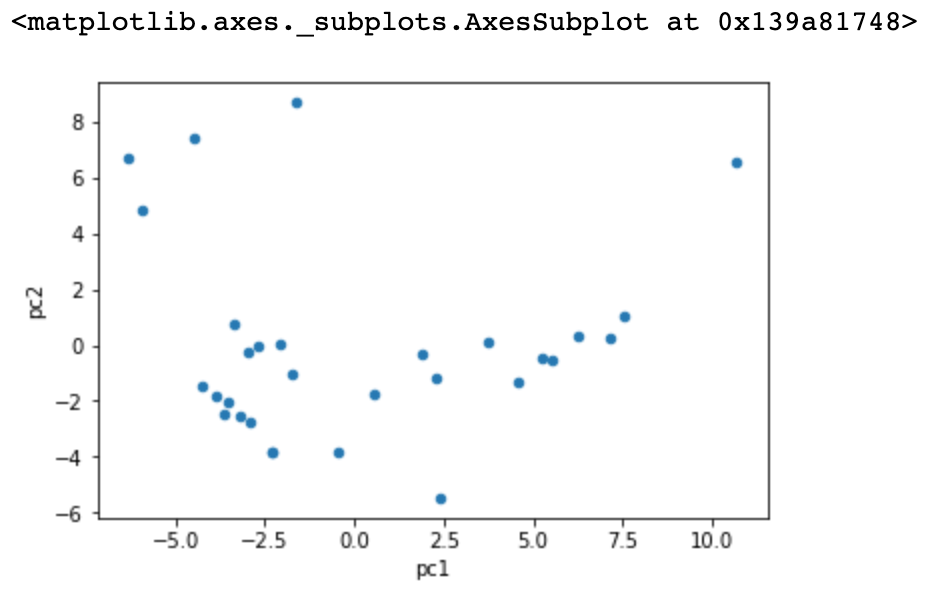Just from this plot alone, we can see some clustering, suggesting that these companies are similar to each other. To verify this, we can add the sector information to each stock and replot the principal components.

``````# add sector information
df_components['sector'] = DJI['Sector']\

# get unique sectors
sectors = list(set(DJI['Sector'].tolist()))

# plot
fig=plt.figure(figsize = (8,8))
ax.set_xlabel('Principal Component 1', fontsize = 12)
ax.set_ylabel('Principal Component 2', fontsize = 12)
cmap = plt.cm.get_cmap('hsv', len(sectors))
for i in range(len(sectors)):
ax.scatter(
x=df_components[df_components['sector'] == sectors[i]]['pc1'],
y=df_components[df_components['sector'] == sectors[i]]['pc2'],
c=cmap(i),
s=50
)
ax.legend(sectors)``````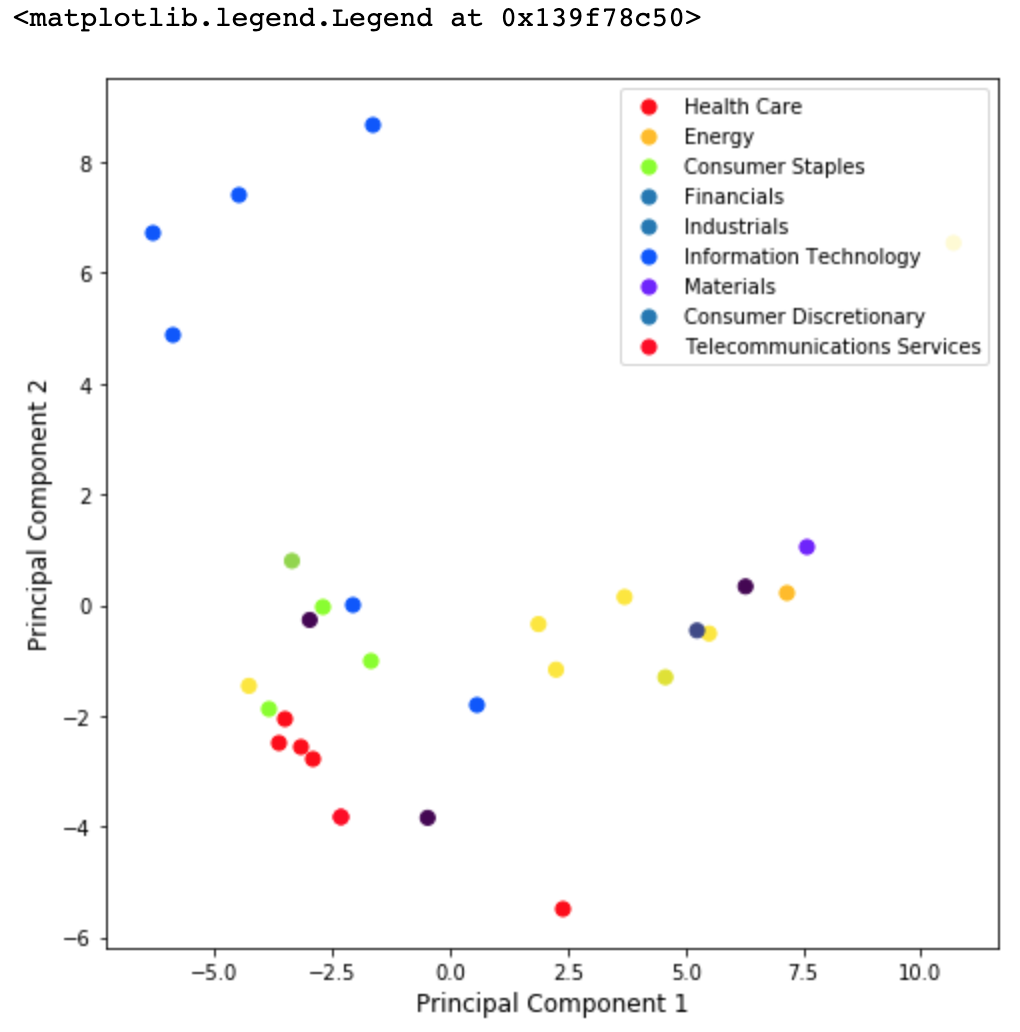We can see that just among these 30 stocks, stocks of the same sector tend to cluster together. This would be even clearer if more stocks were added to the analysis.

Mimicking index returns

I also wanted to see if the principal components generated would be useful in helping me replicate the DJIA returns. To test my hypothesis, I used the first principal component, which has the best explanatory power for the variance in the data and turned the principal component for each stock into a weight. I then fetched the transposed daily returns for the composite stocks and adjusted them by their corresponding weights.

``````weights = abs(df_components['pc1'])/sum(abs(df_components['pc1']))
df_plot = pd.DataFrame(df_daily_returns_trans.loc[DJI_symbols, :])
df_plot['weights'] = weights.tolist()

# iterate through each column except weights
for i in range(df_plot.shape - 1):
df_plot.iloc[:, i] = df_plot.iloc[:, i] * df_plot['weights']

# drop weights column
df_plot = df_plot.drop(['weights'], axis = 1)``````

I plotted them to see how my portfolio would have performed with the calculated weights.

``df_plot.transpose().sum(1).plot()``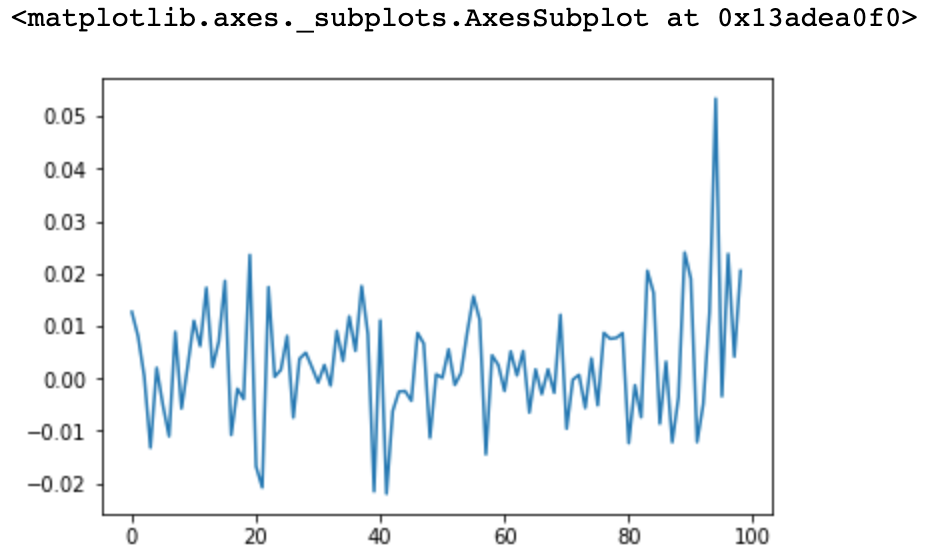I then plotted the actual DIA returns for comparison.

``df_daily_returns[index].plot()``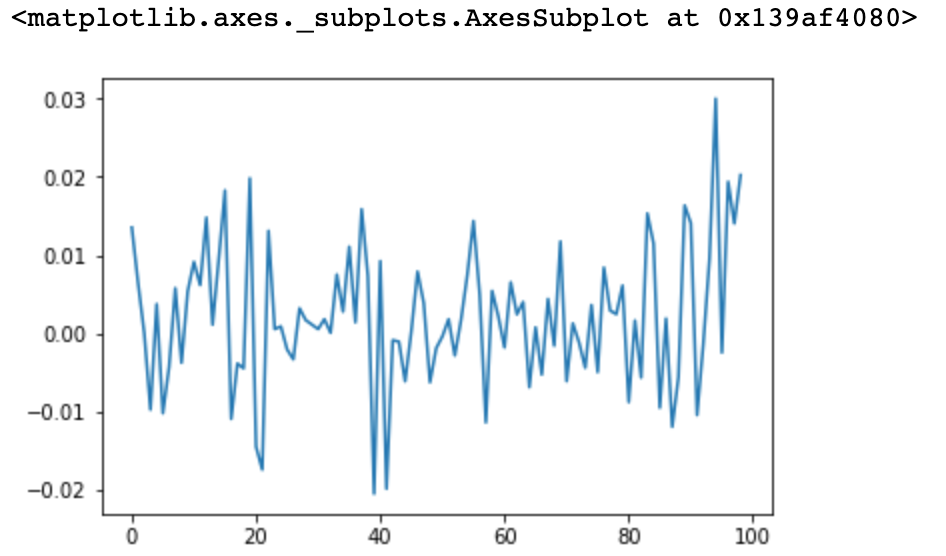Notice how similar the plots are to each other. This means that if I had maintained a portfolio with the stock weights based on PCA, it would have had pretty similar returns to the DIA ETF, and thus the DJIA index.

Published  Feb 20, 2021
Creating a scalable and user-friendly web application for financial analysis using solely Python code.Vanilla Python programs and Jupyter Notebook scripts can be very powerful tools to wrangle, analyze, and visualize data. However, they do not have the best user interfaces. What happens when you want your program to be used by a non-technical user that does not know how to operate command-line tools and shells? Building your program as a web application provides the ability to take in input and display output via an interface that most people would be familiar with.

My web app

In this article, I will be demonstrating how I built a simple web application to retrieve public financial data and calculated an expected share price based on the Discounted Dividend Model (DDM).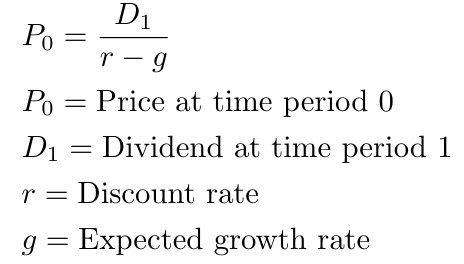My application leveraged the Streamlit framework, which is an open-source framework that enables web applications to be built entirely using Python code. To use Streamlit, you will just need to install the package in your virtual environment:

``pip install streamlit``

Project structure

My project structure for this application is split into three main components: the main file, helper functions, and constants.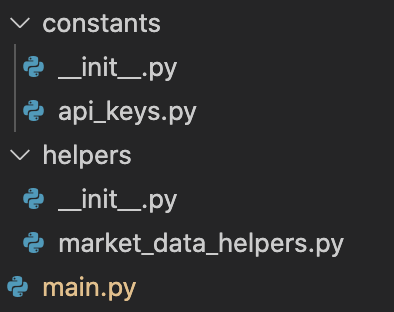This allows for greater readability and scalability of my program code.

Helper functions

As seen in my project structure, I have a file called market_data_helpers.py. The purpose of this script is to maintain functions that would assist with retrieving relevant market data. The following packages will need to be imported at the head of this script to enable the functions to be written to work:

``````import json
import requests``````

I included the retrieve_data function that I had written in a previous article regarding retrieving public financial data via API.

``````def retrieve_data(function: str, symbol: str, api_key: str) -> dict:
"""
Retrieves data from AlphaVantage's open API.
Documentation located at: https://www.alphavantage.co/documentation
"""
# query from API
url = f'https://www.alphavantage.co/query?function={function}&symbol={symbol}&apikey={api_key}'
response = requests.get(url)
data = response.text
# parse output

return parsed``````

Checking the output of the Overview report, I determined that the "Name" and "DividentPerShare" keys contained the information I needed. I then wrote two more functions in market_data_helpers.py to extract the name and dividend per share values.

``````def extract_name(overview) -> str:
return overview['Name']

def extract_dividend_per_share(overview) -> float:
try:
dps = float(overview['DividendPerShare'])
except:
# handle "none"
dps = 0.0
return dps``````

Constants

I created a file called api_keys.py to store my Alpha Vantage API key. This would allow me to retrieve my API key without having to explicitly write it in my main function.

Main

The main.py script puts everything all together.

To use the streamlit framework, the streamlit package has to be imported in the script.

``import streamlit as st``

I also imported my Alpha Vantage API key and the helper functions written above.

``````from constants.api_keys import API_KEYS
from helpers.market_data_helpers import retrieve_data, extract_dividend_per_share, extract_name``````

A function called ddm was created to execute the DDM logic based on the given formula above.

``````def ddm(dividend: float, r: float, g: float) -> float:
return (dividend * (1 + g))/(r - g)``````

Finally, I implemented the main function which would dictate how the web application works. I used the text_input and number_input methods from the Streamlit library to receive ticker, expected growth rate, and discount rate values from the user.

``````ticker = st.text_input('Please input ticker of stock you wish to analyze')
g = st.number_input('Expected annual growth')
r = st.number_input('Discount rate')``````

I then created a button using the button method in the Streamlit library.

``calculate_button = st.button('Calculate DDM')``

The value returned from this button will be false by default, but will become true once it is clicked. I could then have my program check if the button was clicked by checking the boolean value returned, and execute the DDM analysis if the value is true.

The execution of the analysis is as follows:

1. Retrieve the Overview report of the company associated with the given ticker using the retrieve_data helper function.
2. Extract the name and dividend per share within the Overview report using the extract_name and extract_dividend_per_share helper functions.
3. Calculate the expected price based on the DDM model using the ddm function created earlier.

The entirety of the main.py script is as follows:

``````import streamlit as st
from constants.api_keys import API_KEYS
from helpers.market_data_helpers import retrieve_data, extract_dividend_per_share, extract_name

def main():
st.title('Welcome to Fundamentals Analyzer')

ticker = st.text_input('Please input ticker of stock you wish to analyze')
g = st.number_input('Expected annual growth')
r = st.number_input('Discount rate')

calculate_button = st.button('Calculate DDM')

if calculate_button:
if ticker == '':
else:
overview = retrieve_data('OVERVIEW', ticker, API_KEYS['alpha_vantage'])

name = extract_name(overview)
dividend = extract_dividend_per_share(overview)

st.subheader('Expected Share Price (based on DDM)')
st.write(str(round(ddm(dividend, r, g), 2)))

def ddm(dividend: float, r: float, g: float) -> float:
return (dividend * (1 + g))/(r - g)

if __name__ == '__main__':
main()
``````

Deploying the application

Once the code is completed, the application can be deployed locally by navigating to the project folder in the command line and running it using Streamlit:

``streamlit run main.py``

The web application should then be launched on localhost and it can be viewed in a browser.